# 深入理解 Cookie 的 SameSite 属性

``````// 服务端
const http = require("http");

http
.createServer((req, res) => {
if (req.url == "/") {
res.end("hello world");
} else if (req.url == "/favicon.ico") {
res.statusCode = 204;
res.end();
} else {
]);
res.end("some data");
}
})
.listen(80);

``````// 客户端
var xhr = new XMLHttpRequest();
xhr.open('GET', "/someapi");
xhr.send();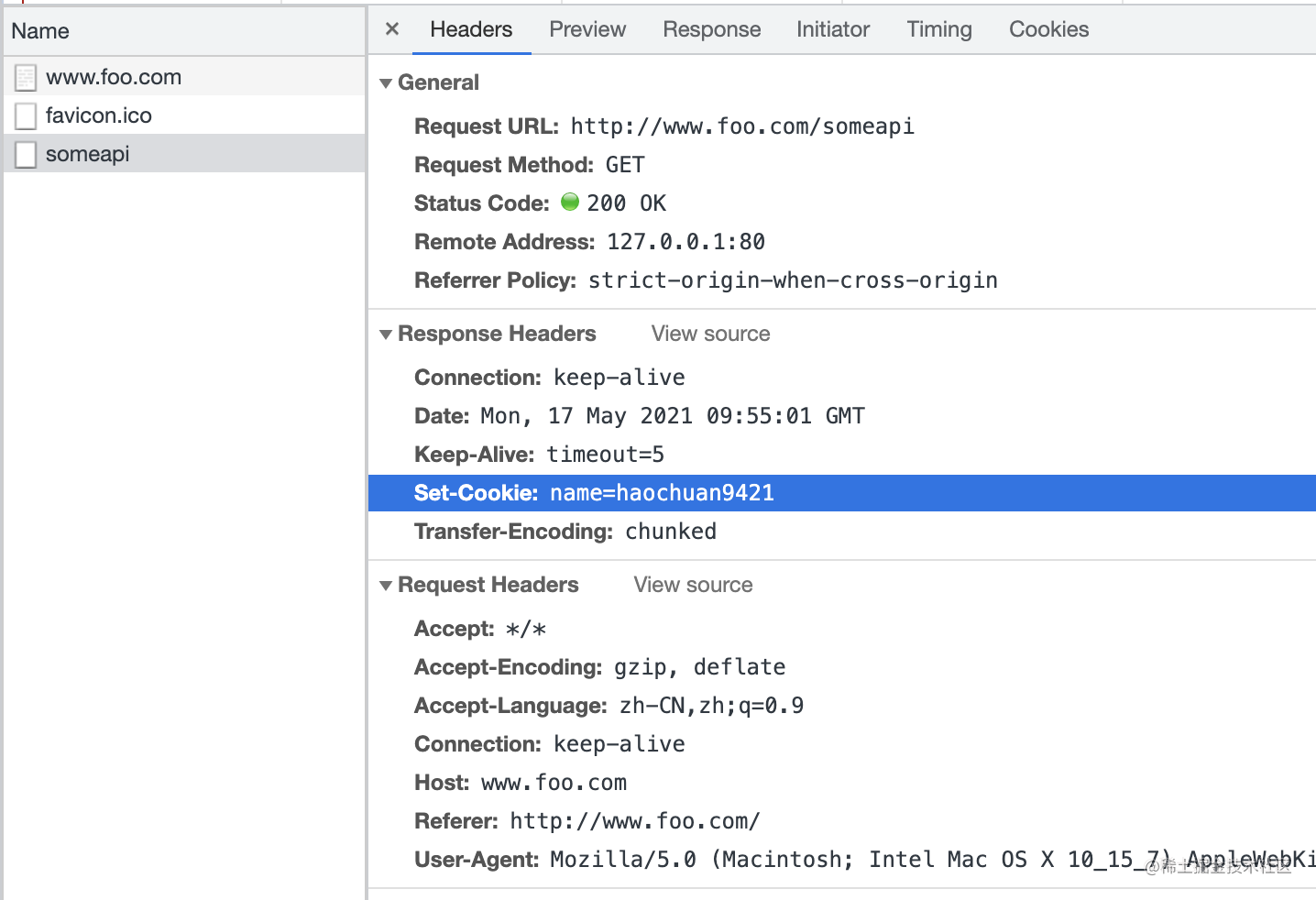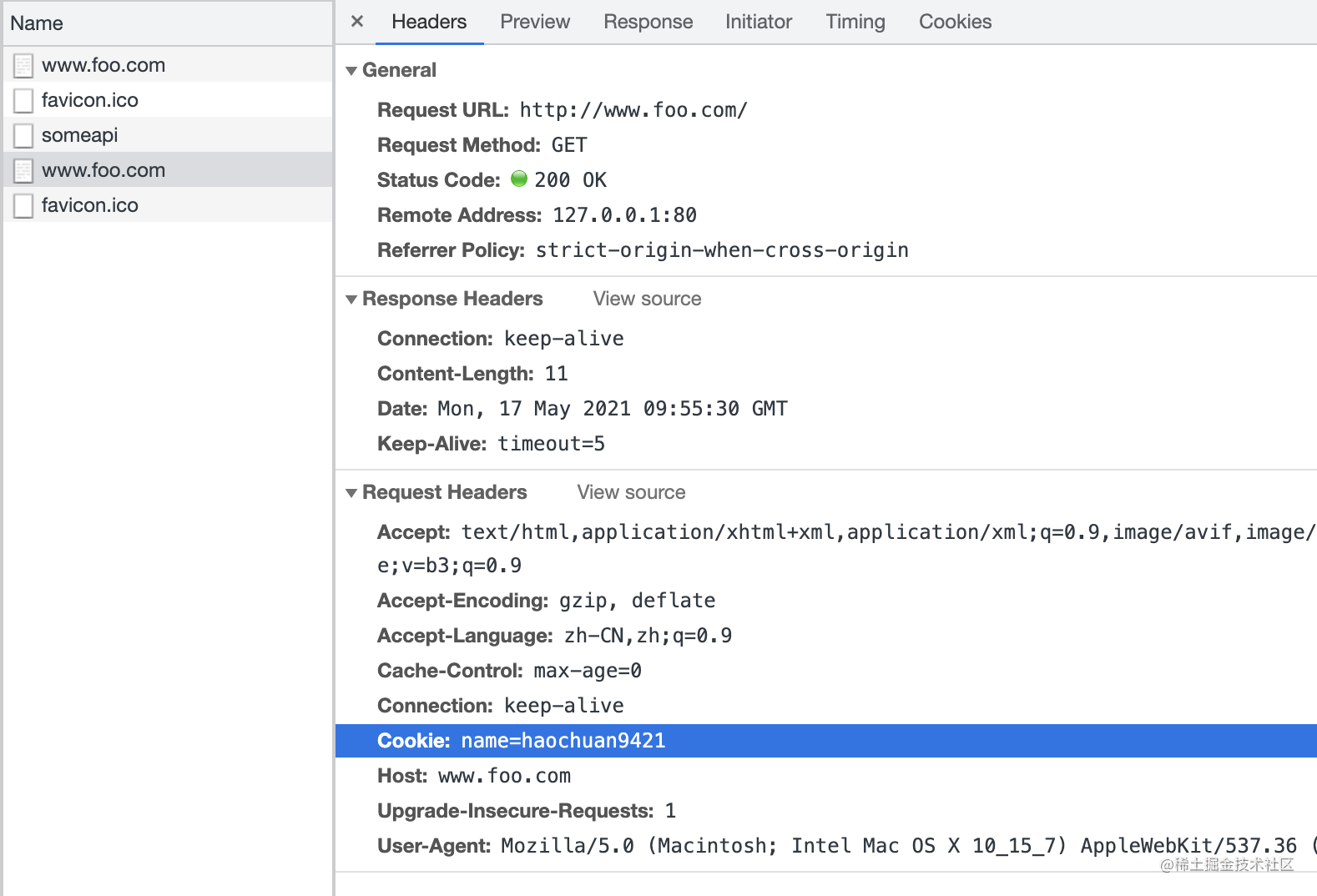### SameSite 属性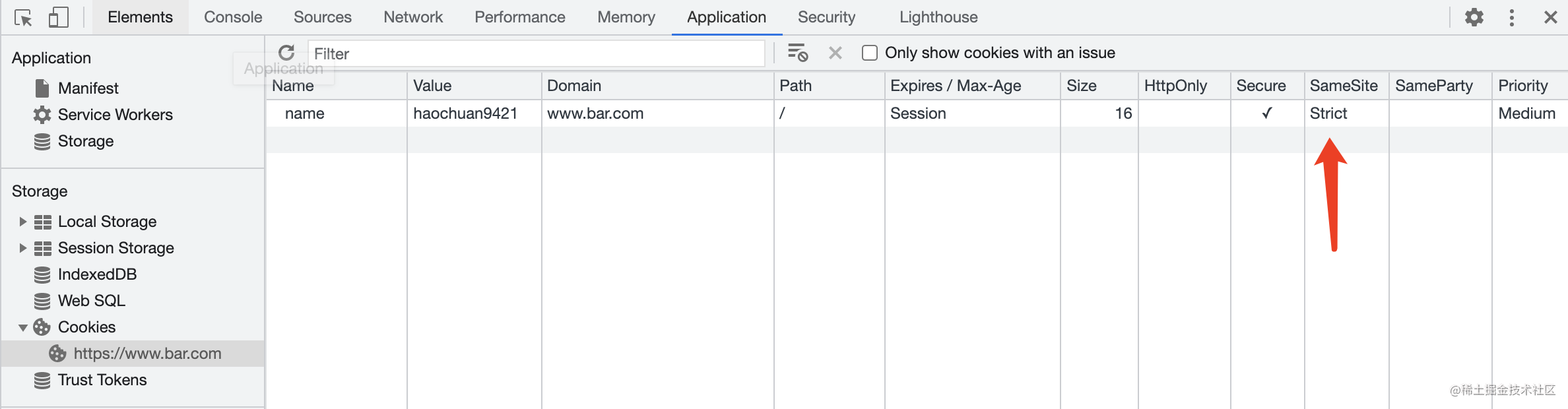`SameSite` 有三个可选值：

• `Strict`
• `Lax`
• `None`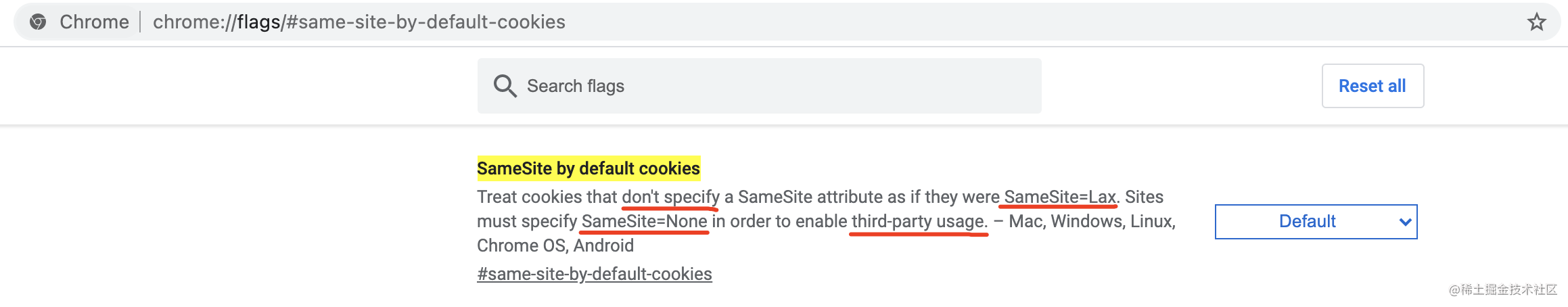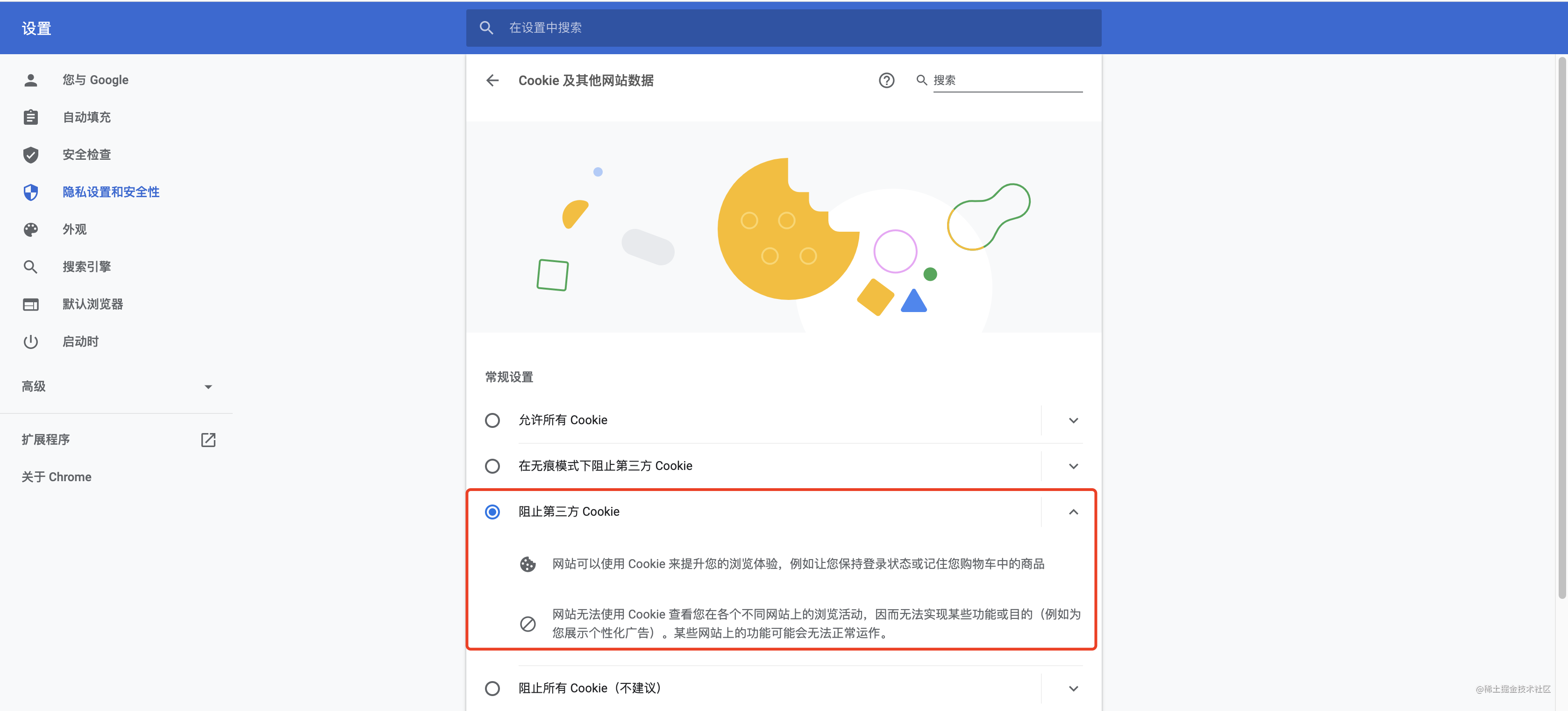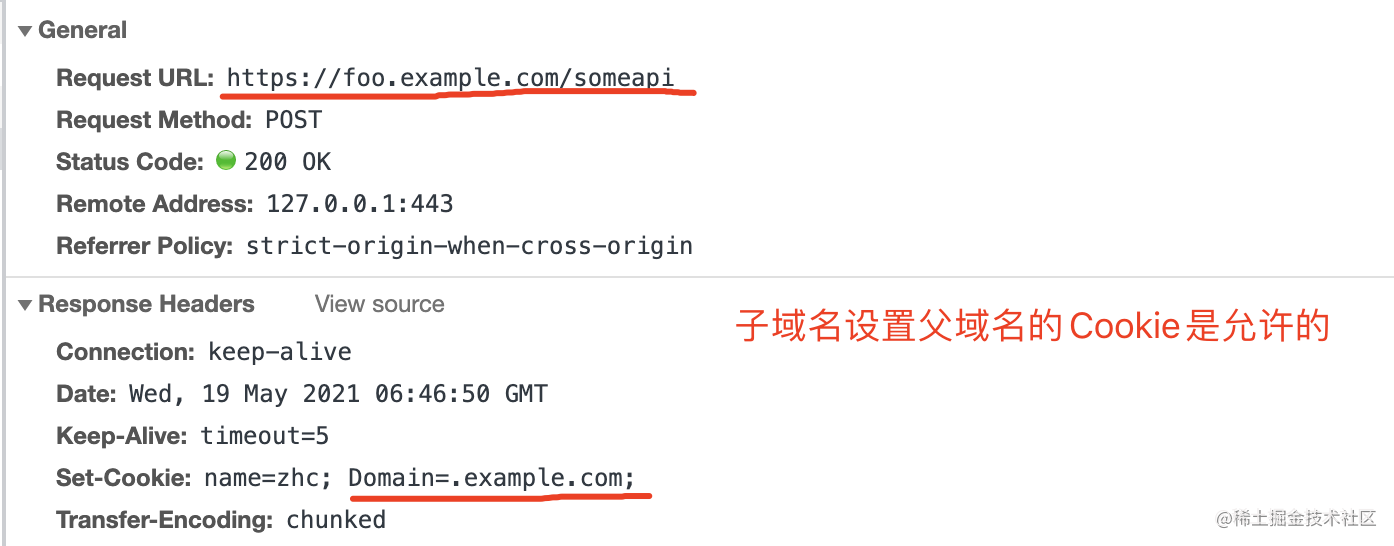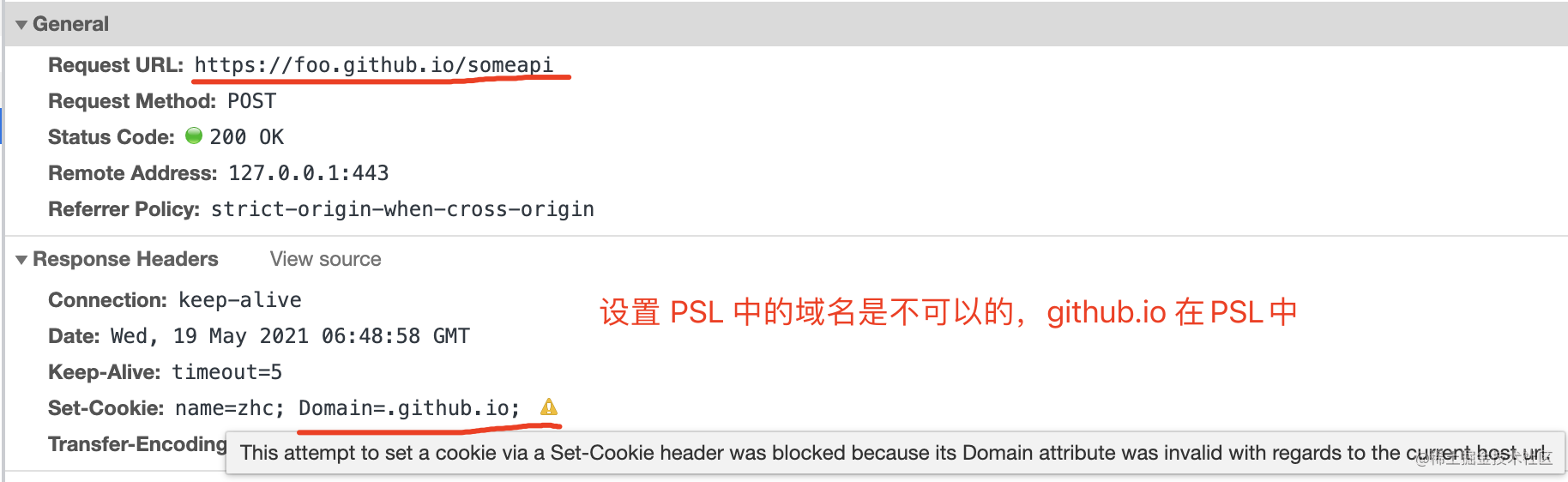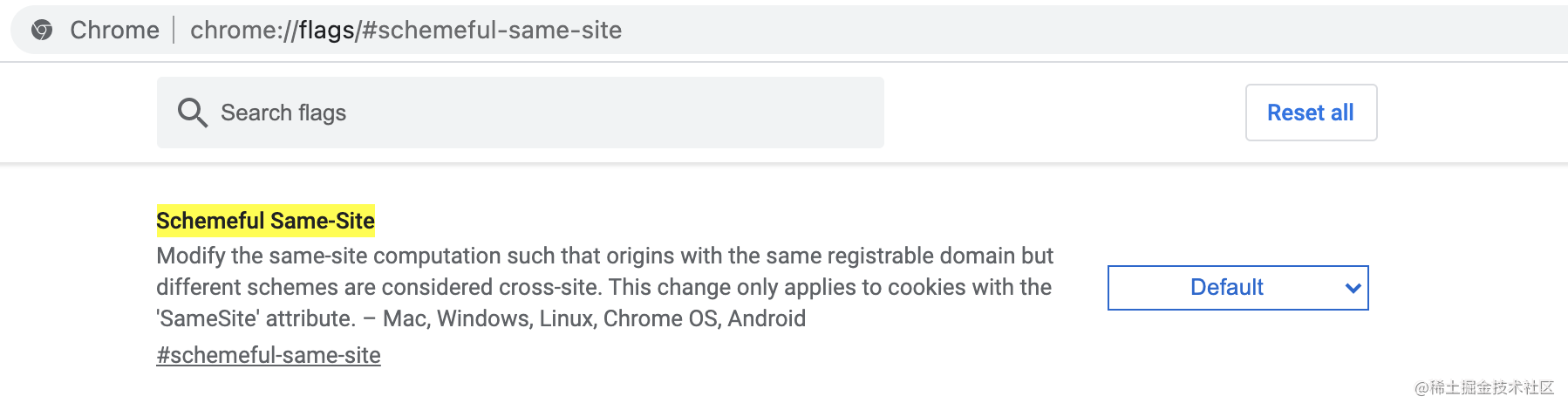### 1. AJAX 请求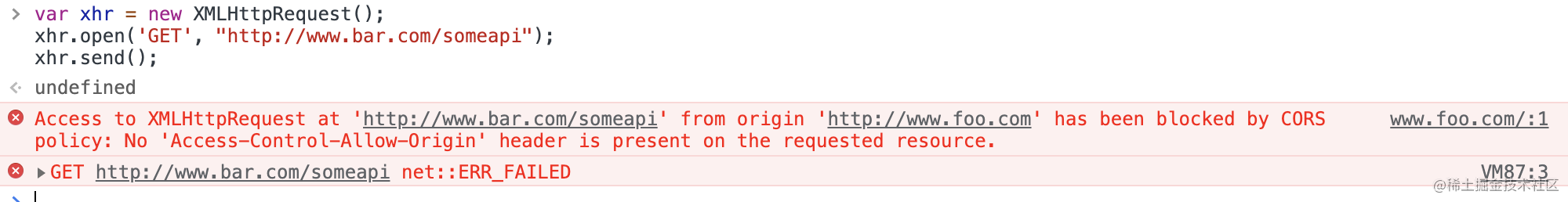``````const http = require("http");

http
.createServer((req, res) => {
if (req.url == "/") {
res.end("hello world");
} else if (req.url == "/favicon.ico") {
res.statusCode = 204;
res.end();
} else {
["Access-Control-Allow-Origin", "*"], // 允许跨域请求
]);
res.end("some data");
}
})
.listen(80, "0.0.0.0");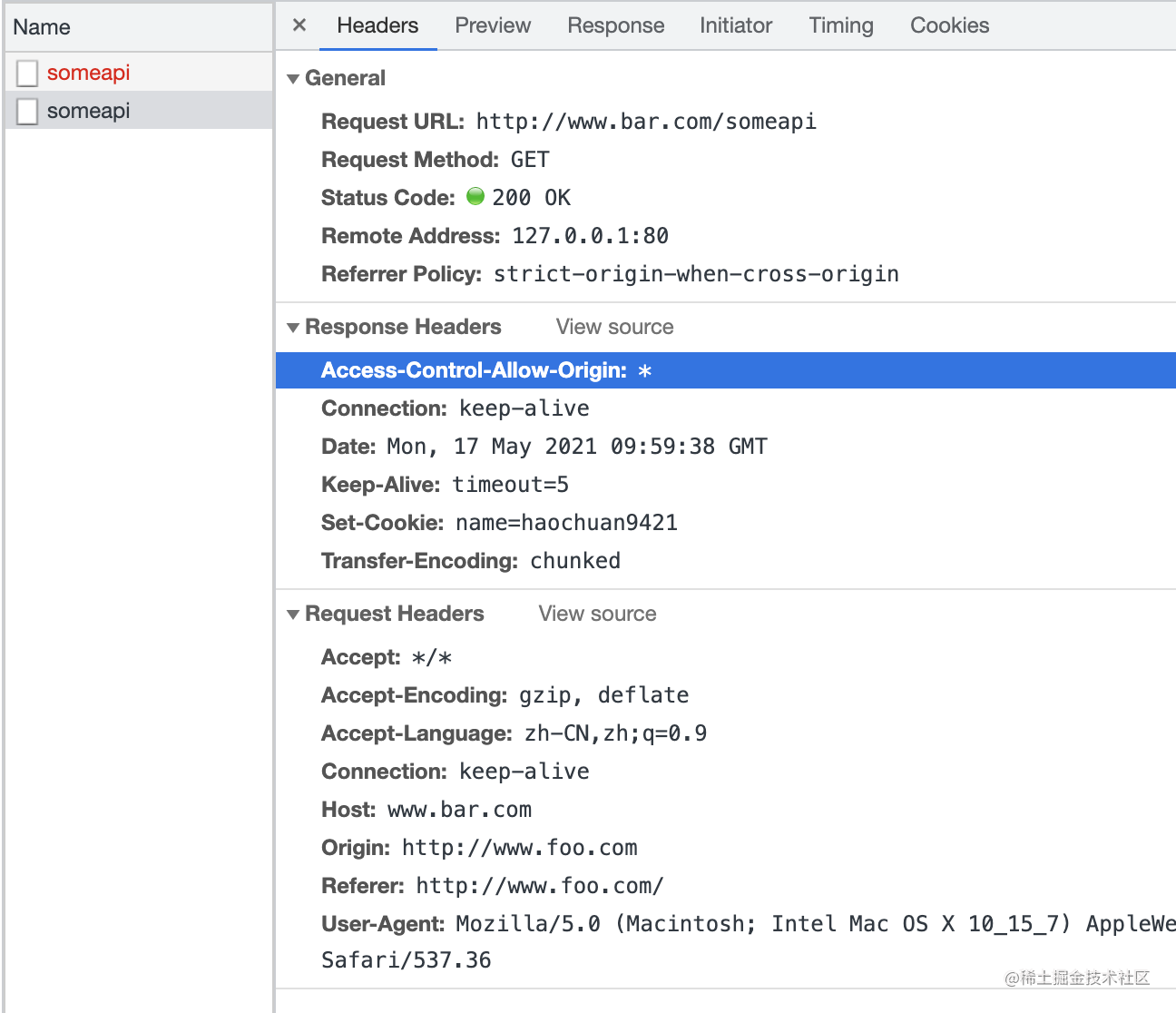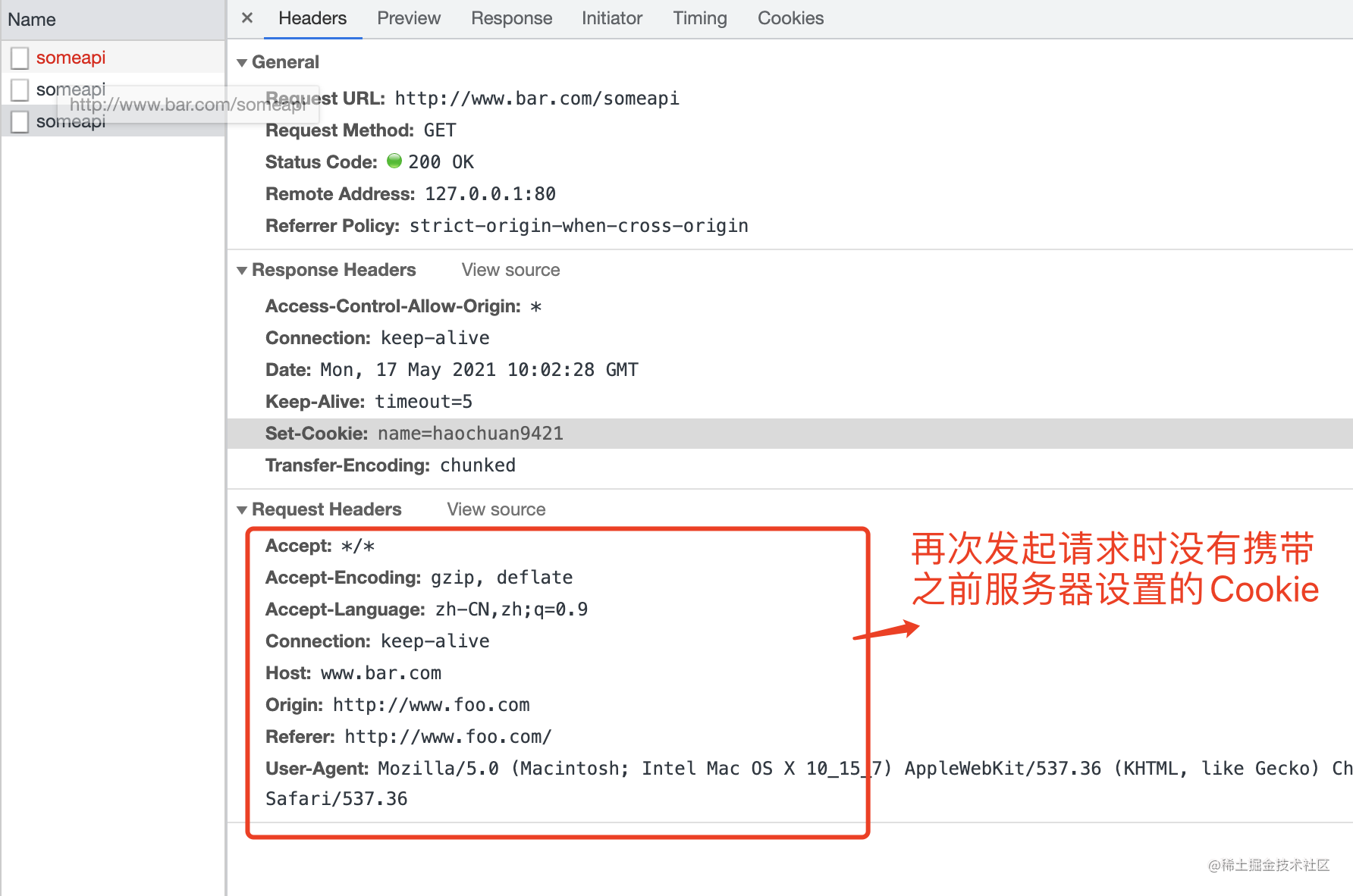1. 网站开启 https 并将 Cookie 的 Secure 属性设置为 true
2. Access-Control-Allow-Origin 设置为具体的 origin，而不是 *
3. Access-Control-Allow-Credentials 设置为 true
4. SameSite 属性设置为 None

``````const https = require("https");
const fs = require("fs");

https
.createServer(
{
},
(req, res) => {
if (req.url == "/") {
res.end("hellow world");
} else if (req.url == "/favicon.ico") {
res.statusCode = 204;
res.end();
} else {
? [
]
: []),
]);
res.end("some data");
}
}
)
.listen(443, "0.0.0.0");

``````var xhr = new XMLHttpRequest();
xhr.withCredentials = true;
xhr.open('GET', "https://www.bar.com/someapi");
xhr.send();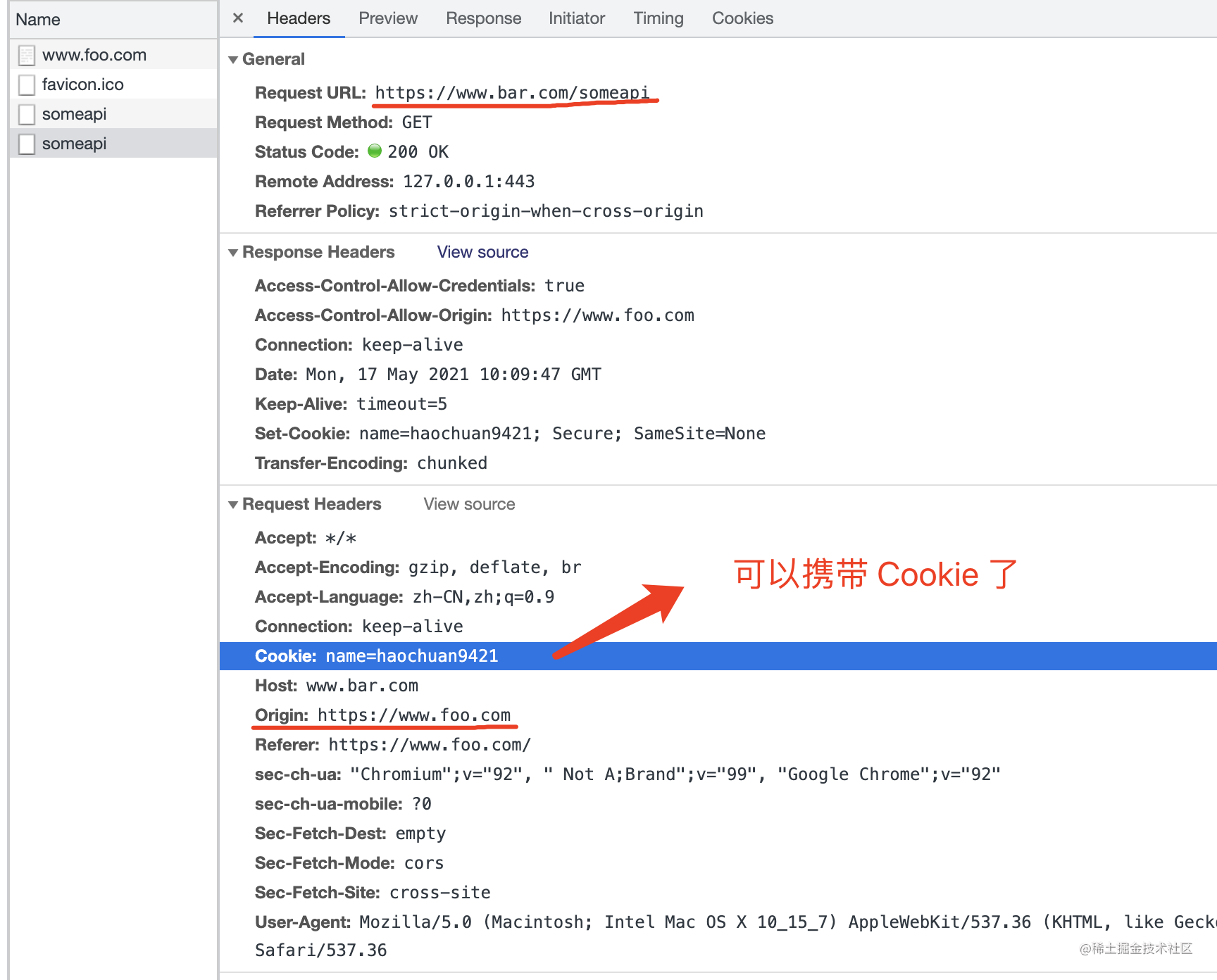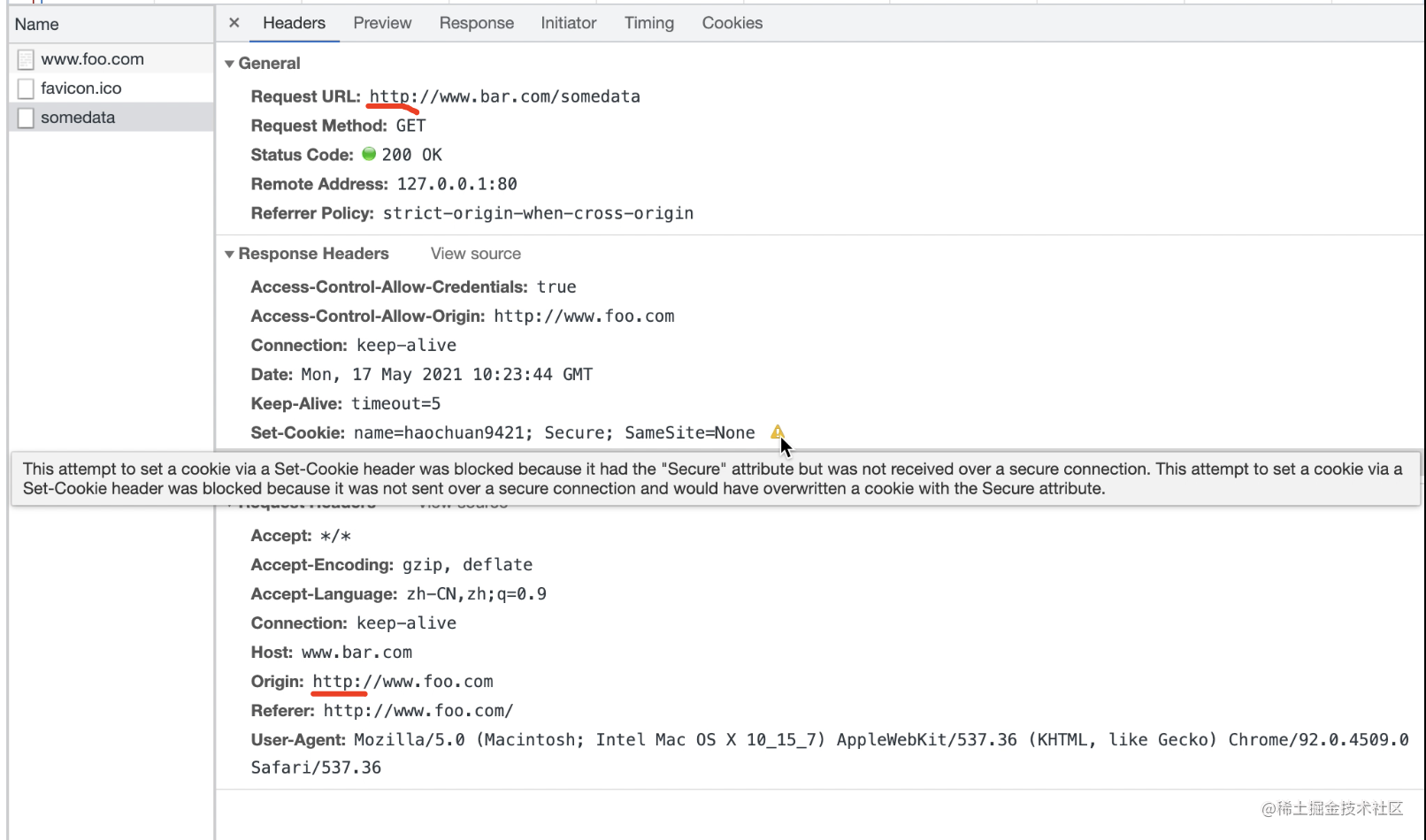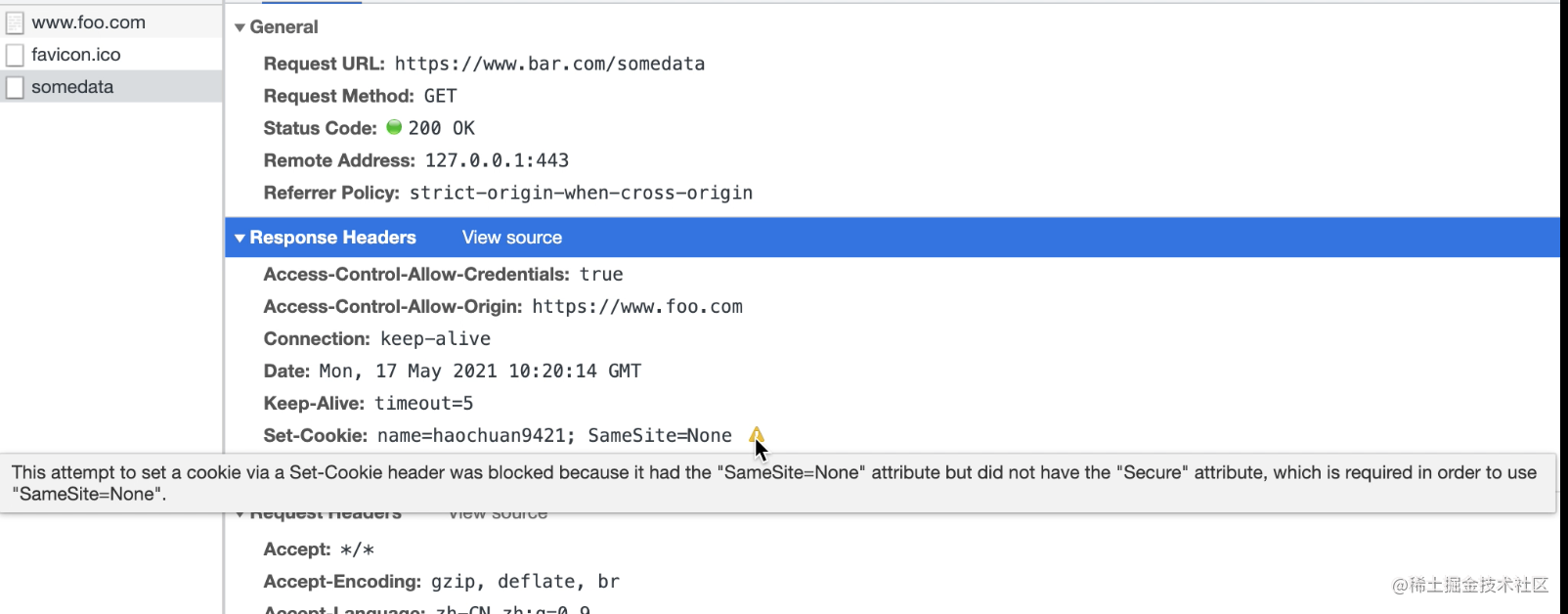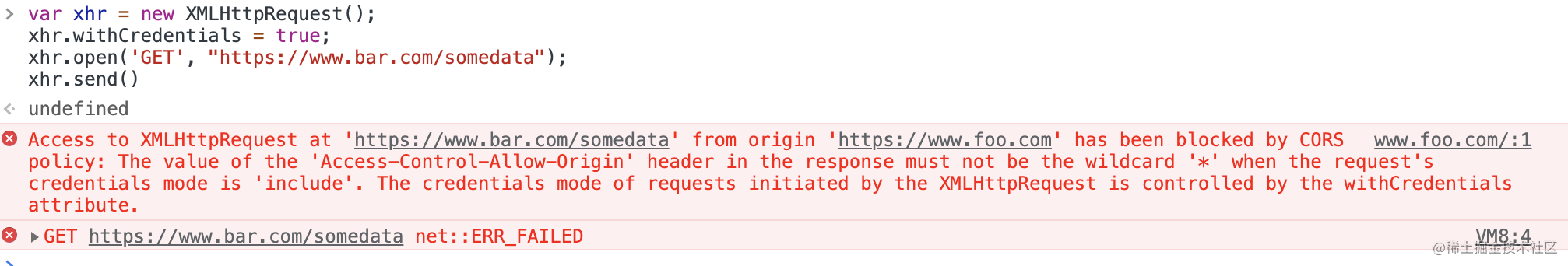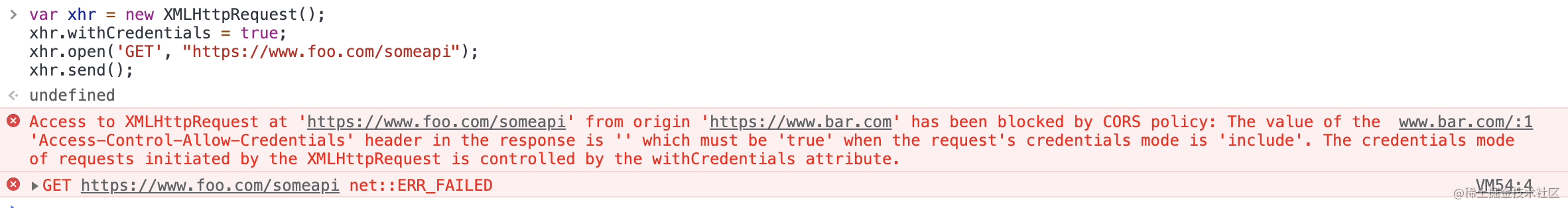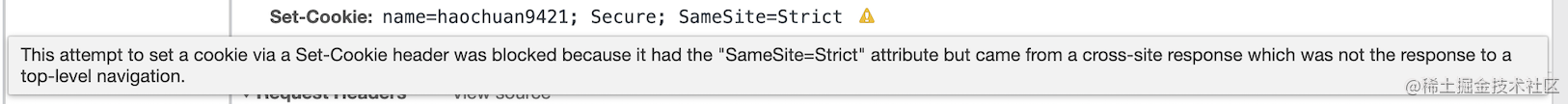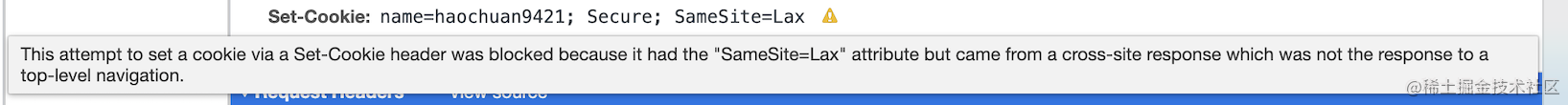``````fetch("https://www.bar.com/somedata", {
"method": "GET",
"credentials": "include"
})

### 2. 嵌套第三方 iframe

``````const https = require("https");
const fs = require("fs");

https
.createServer(
{
},
(req, res) => {
if (req.url == "/") {
res.end(`<div>这是父页面</div>
<iframe src="https://www.bar.com/"></iframe>`);
} else {
["Content-Type", "text/html;charset=utf-8"],
]);
res.end(`<div>这是子页面</div>`);
}
} else {
res.statusCode = 204;
res.end();
}
}
)
.listen(443, "0.0.0.0");

``````<iframe
src="//player.bilibili.com/player.html?bvid=BV1Vv41157uK&high_quality=1"
allowfullscreen="allowfullscreen"
width="100%"
height="500"
scrolling="no"
frameborder="0"
></iframe>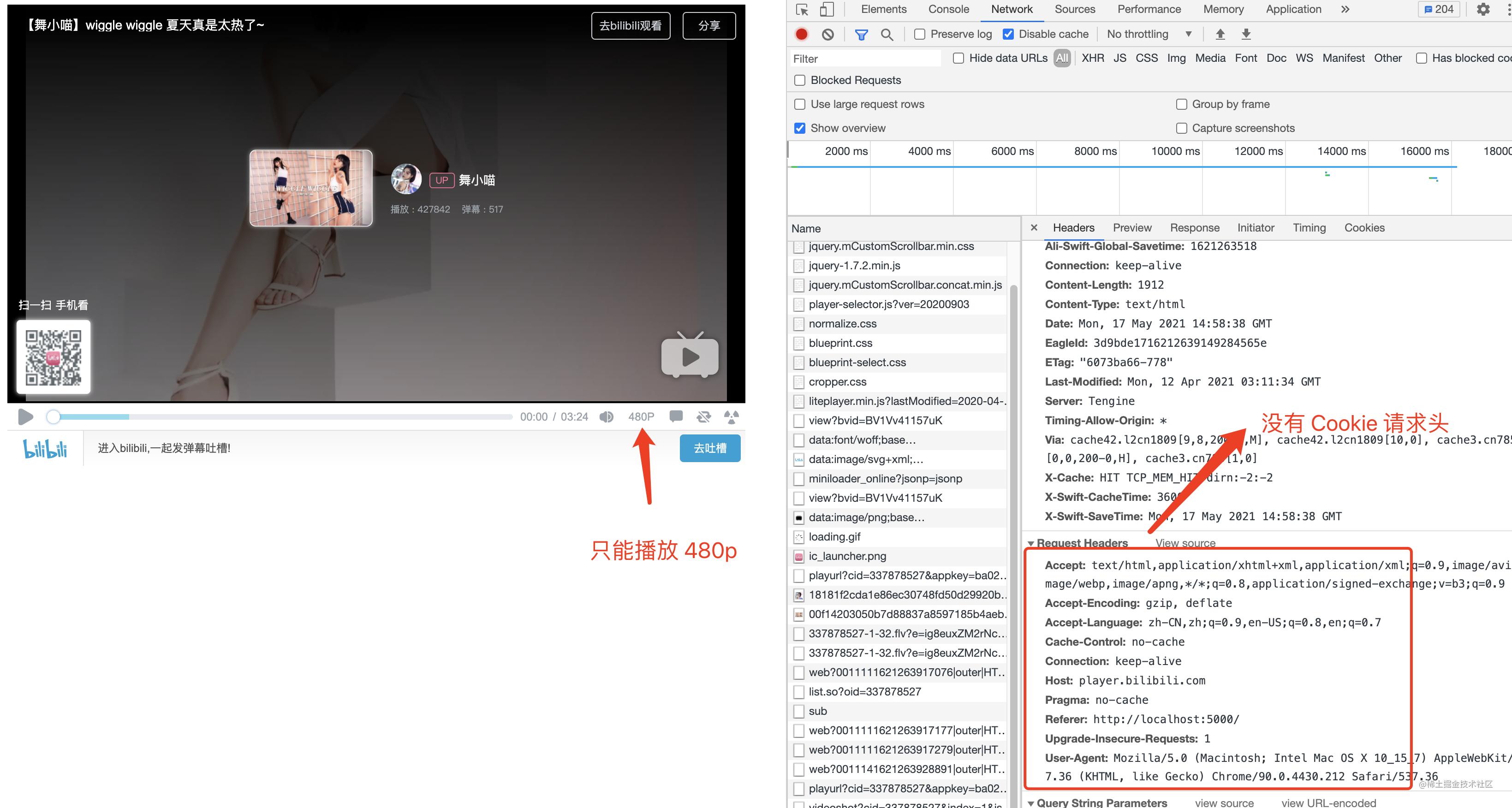### 3. 加载第三方图片或脚本等

``````const https = require("https");
const fs = require("fs");

https
.createServer(
{
},
(req, res) => {
if (req.url == "/") {
res.end(`<div>这是父页面</div>
<img src="https://www.bar.com/"></img>`);
} else {
["Content-Type", "image/png"],
]);
}
} else {
res.statusCode = 204;
res.end();
}
}
)
.listen(443, "0.0.0.0");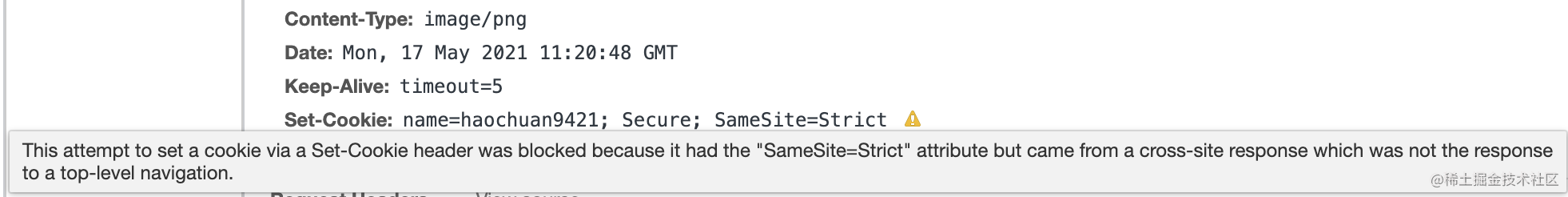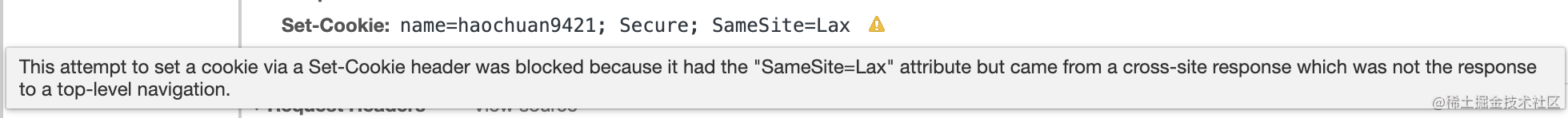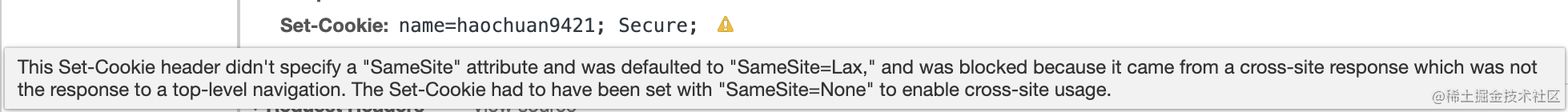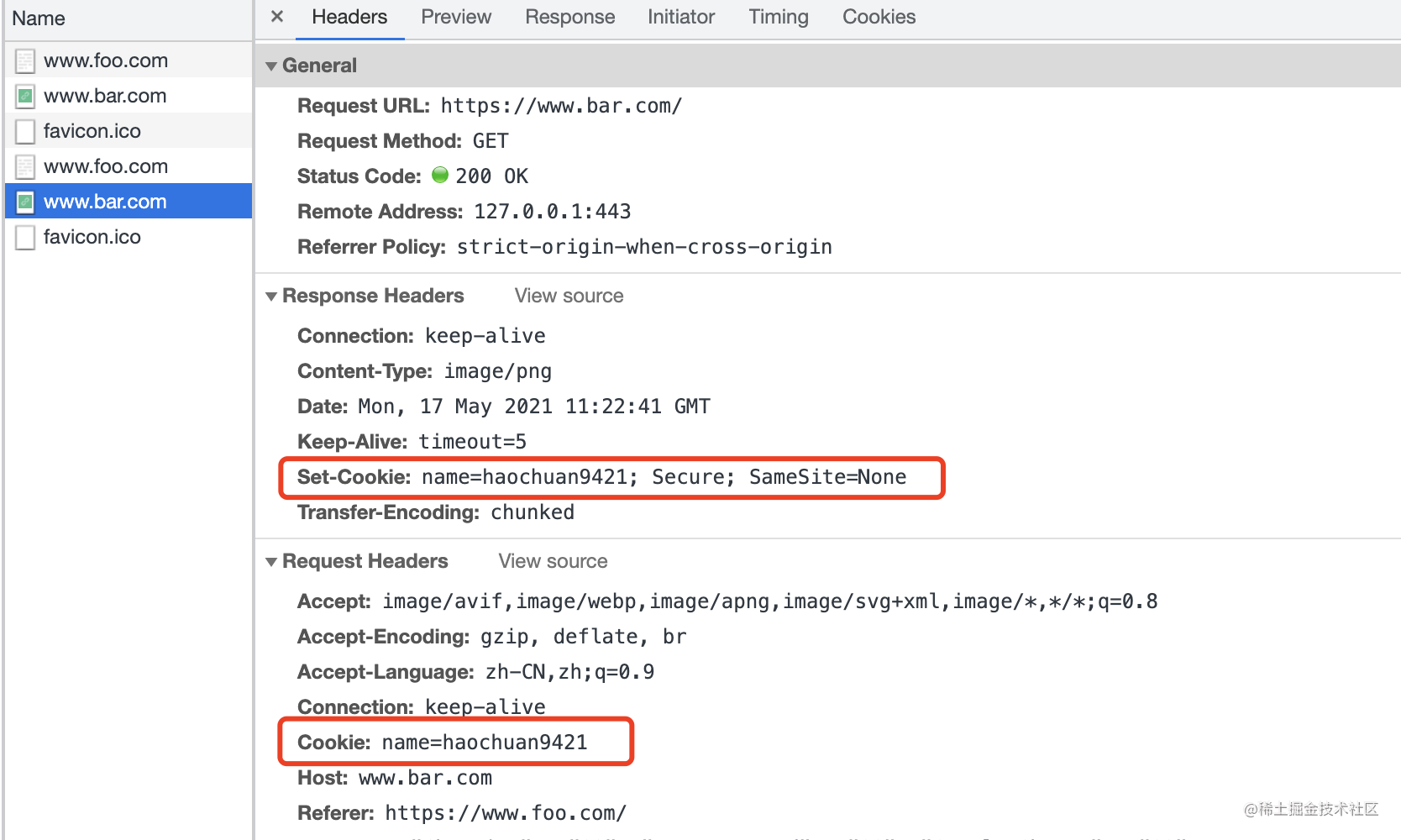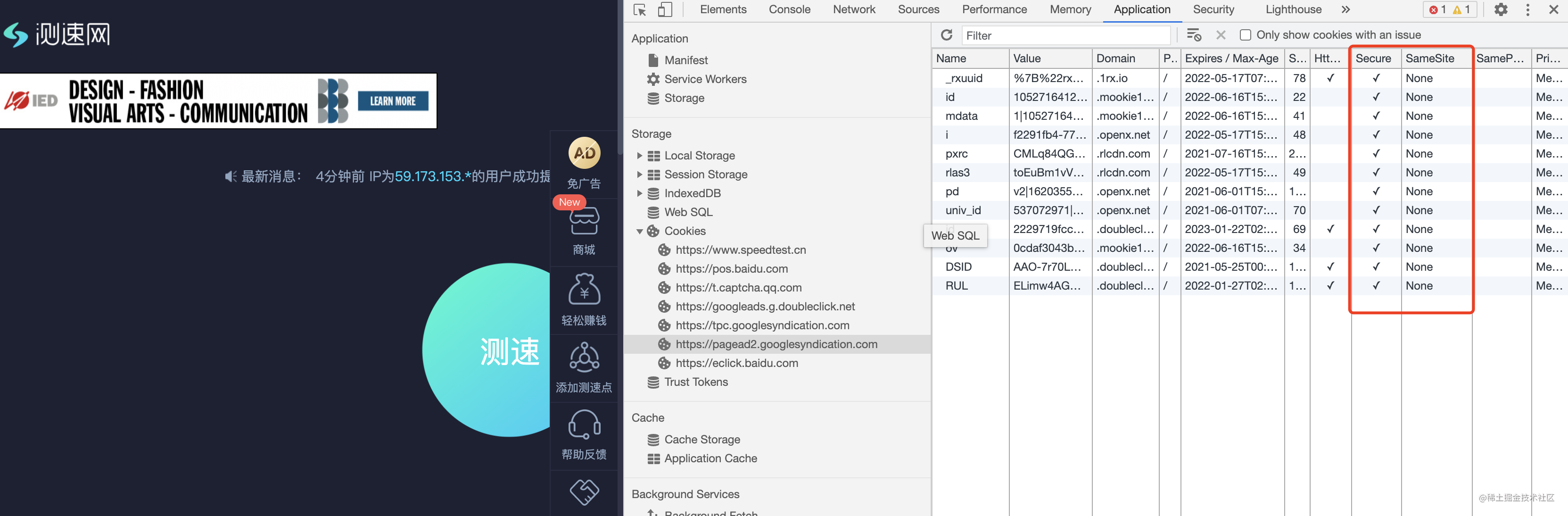### 4. 提交表单到第三方网站

``````const https = require("https");
const fs = require("fs");

https
.createServer(
{
},
(req, res) => {
if (req.url == "/") {
res.end(`<form action="https://www.bar.com/" method="post" enctype="multipart/form-data">
<input type="text" name="name" />
<input type="number" name="age" />
<button type="submit">提交</button>
</form>`);
} else {
]);
res.end("ok");
}
} else {
res.statusCode = 204;
res.end();
}
}
)
.listen(443, "0.0.0.0");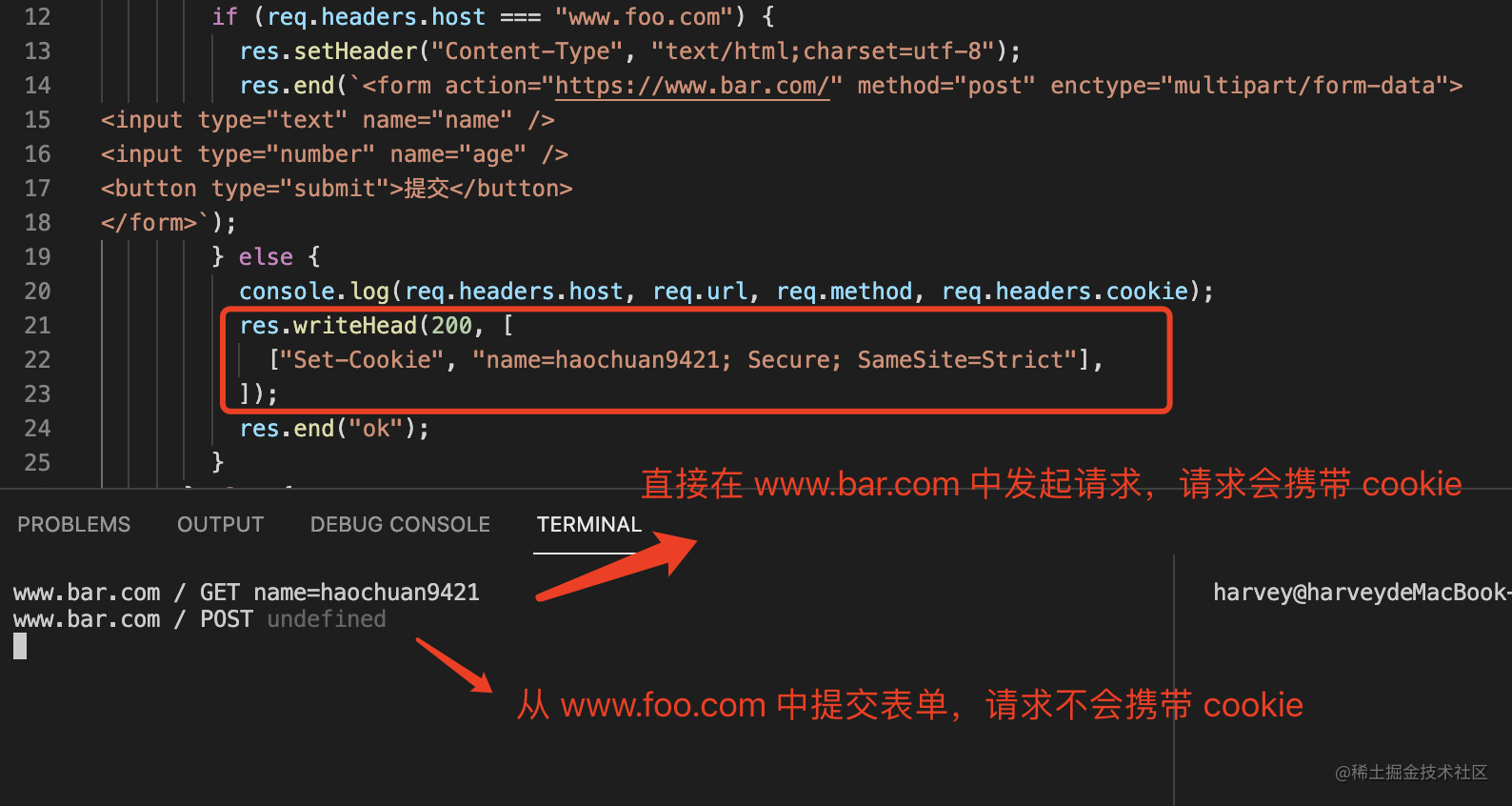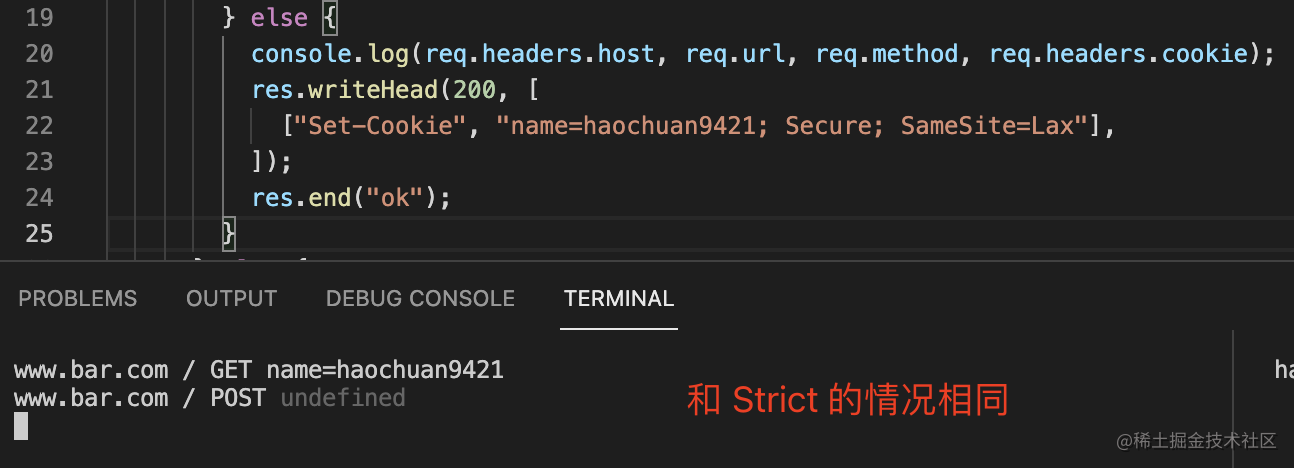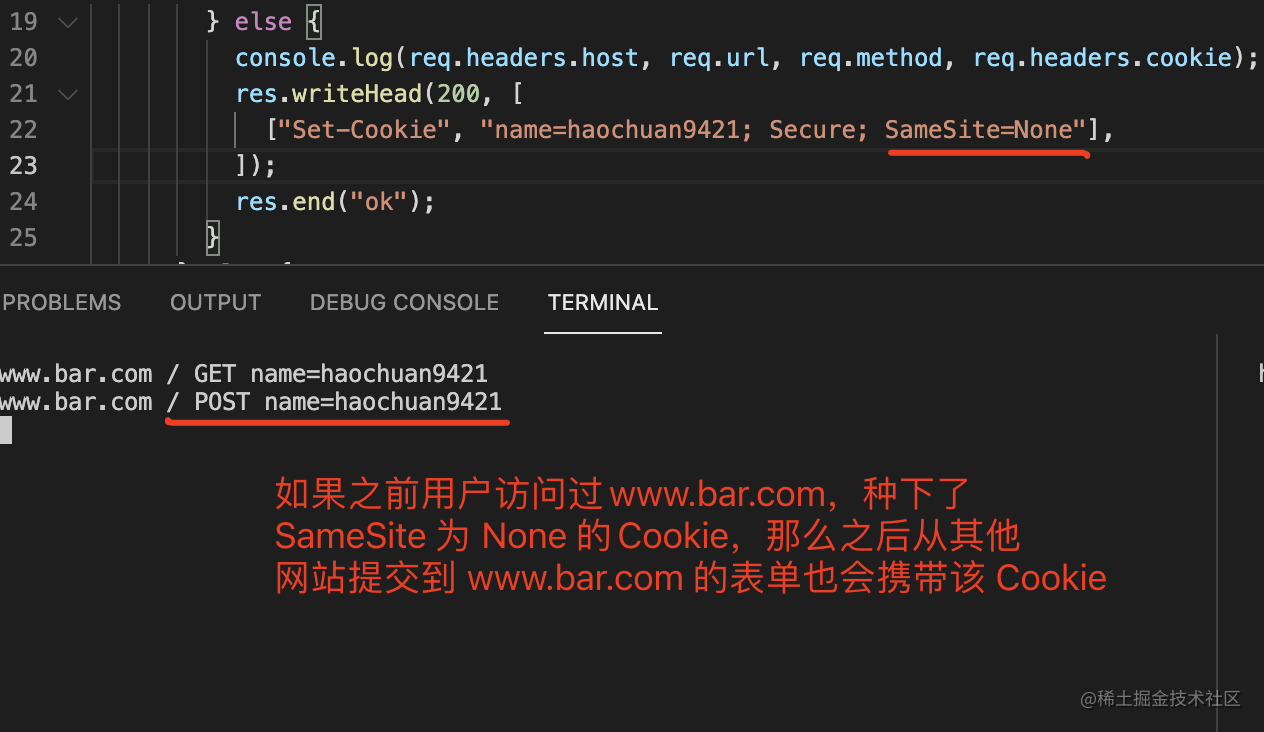``````// 这是我们自己正常的网站
const https = require("https");
const fs = require("fs");

https
.createServer(
{
},
(req, res) => {
if (req.url == "/") {
// 我们网站首页有一个转账的表单
res.end(`<form action="/transfer" method="post">
<input type="number" name="money" />
<button type="submit">提交</button>
</form>`);
} else if (req.url == "/login") {
} else if (req.url == "/transfer") {
// 登录后的用户可以转账，未登录的不能转账
} else {
res.statusCode = 204;
res.end();
}
}
)
.listen(443, "0.0.0.0");

``````// 这是一个要伪造请求的恶意网站
const https = require("https");
const fs = require("fs");

https
.createServer(
{
},
(req, res) => {
if (req.url == "/") {
res.end(`<div>这是一个恶意网站</div>
<form
id="fake-form"
action="https://www.bar.com/transfer"
method="post"
target="submit-target"
>
<input type="hidden" name="money" value="1000" />
</form>
<iframe name="submit-target"></iframe>
<script>document.getElementById("fake-form").submit();</script>`);
} else {
res.statusCode = 204;
res.end();
}
}
)
.listen(443, "0.0.0.0");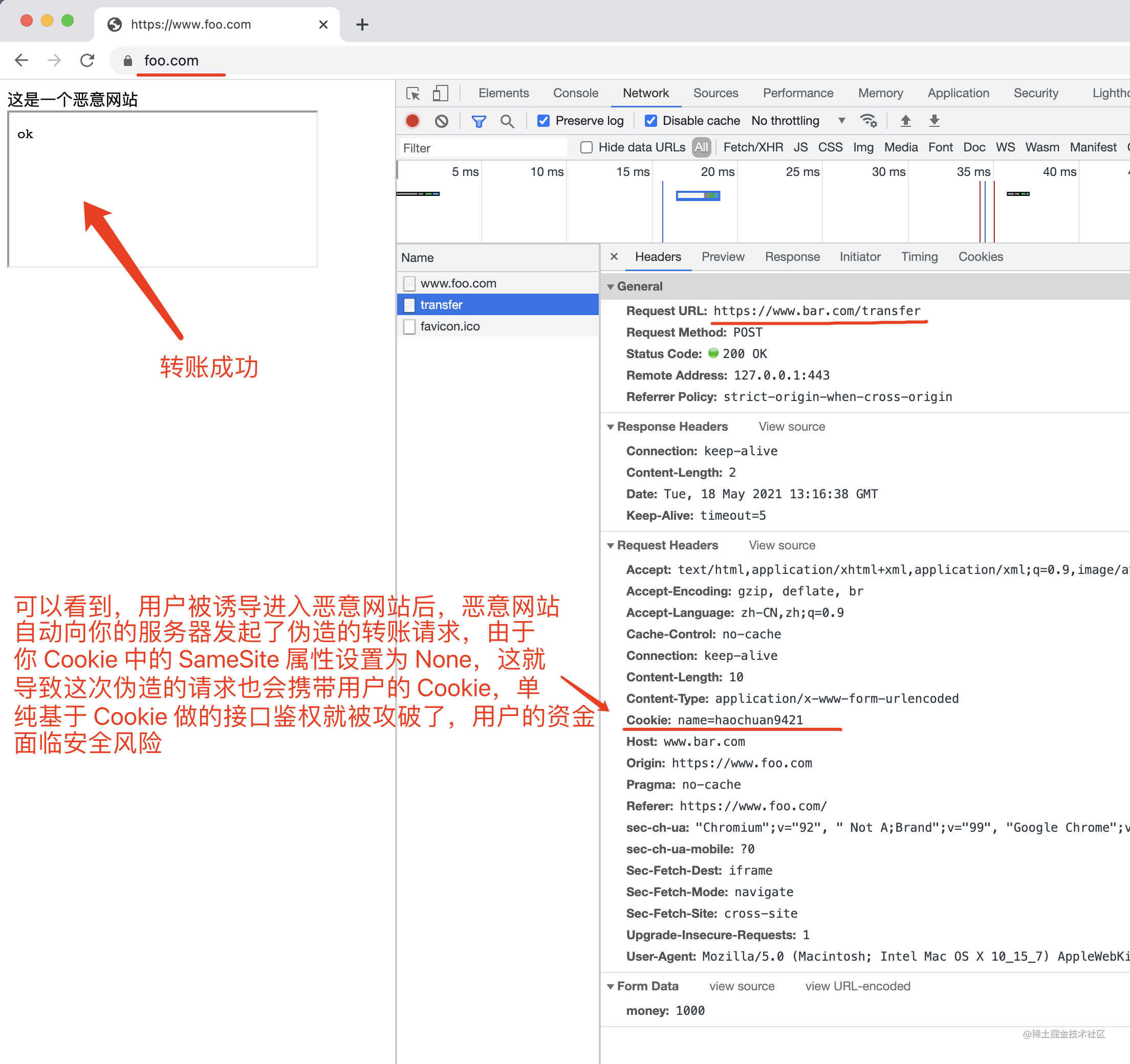### 5. 链接跳转第三方网站

``````const https = require("https");
const fs = require("fs");

https
.createServer(
{
},
(req, res) => {
if (req.url == "/") {
res.end(`<div>foo page</div>
<a href="https://www.bar.com/">www.bar.com</a>`);
} else {
["Content-Type", "text/html;charset=utf-8"],
]);
res.end("bar page");
}
} else {
res.statusCode = 204;
res.end();
}
}
)
.listen(443, "0.0.0.0");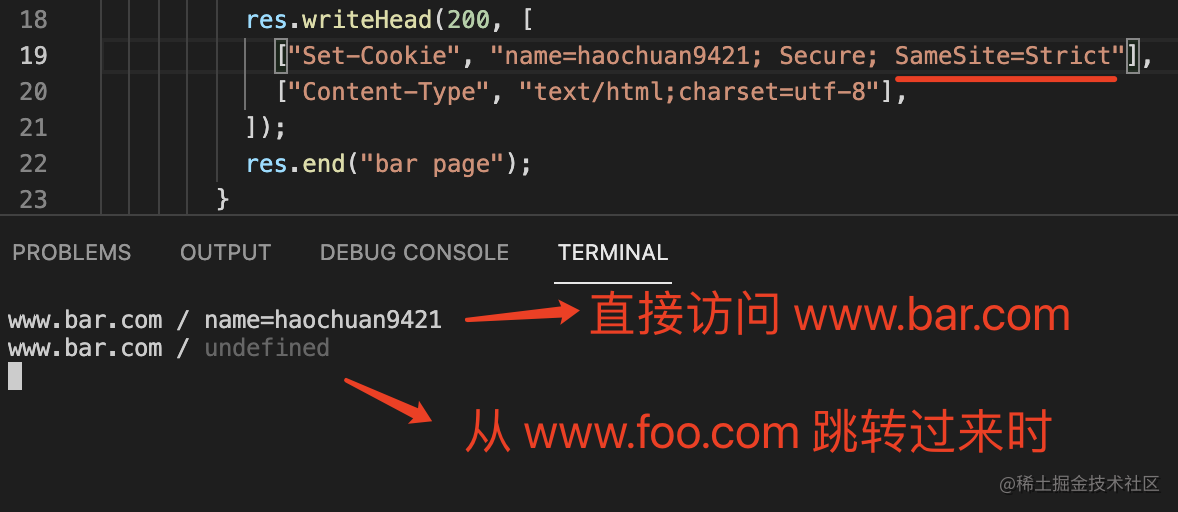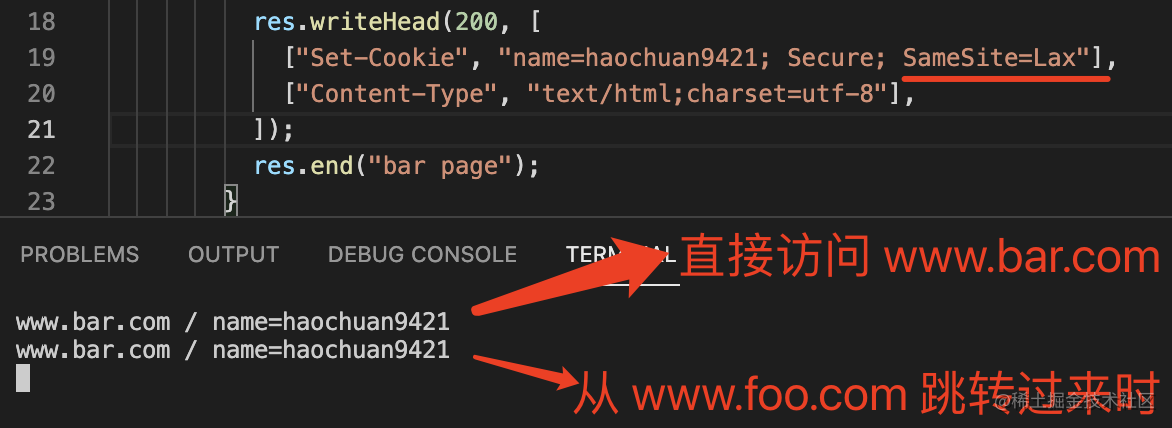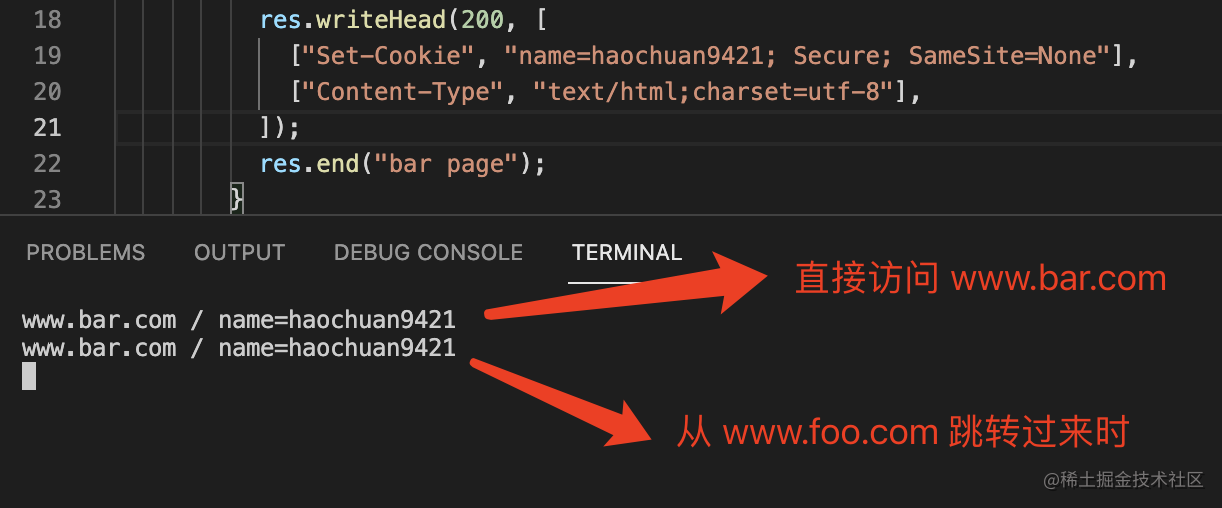`Strict ` 这个规则过于严格，可能造成非常不好的用户体验。比如，当前网页有一个 GitHub 链接，用户点击跳转就不会带有 GitHub 的 Cookie，跳转过去总是未登陆状态。

### 总结

`Strict` 最为严格，表示完全禁止“第三方 Cookie”，只有当前网页的 URL 与请求目标一致时，才会带上 Cookie，一般用于保证系统的封闭性和安全性。

`Lax` 是目前大多数现代浏览器的默认值，他在保证安全性的前提下，也可以避免一些不好的用户体验，比如从别的网站跳转过时会没有登录态。

`None` 是最为宽松的一种设定，通常用于开放我们的服务给不同的第三方接入，同时又需要追踪用户的场景，比如广告，设置为 `None` 时需要考虑开放的安全性。